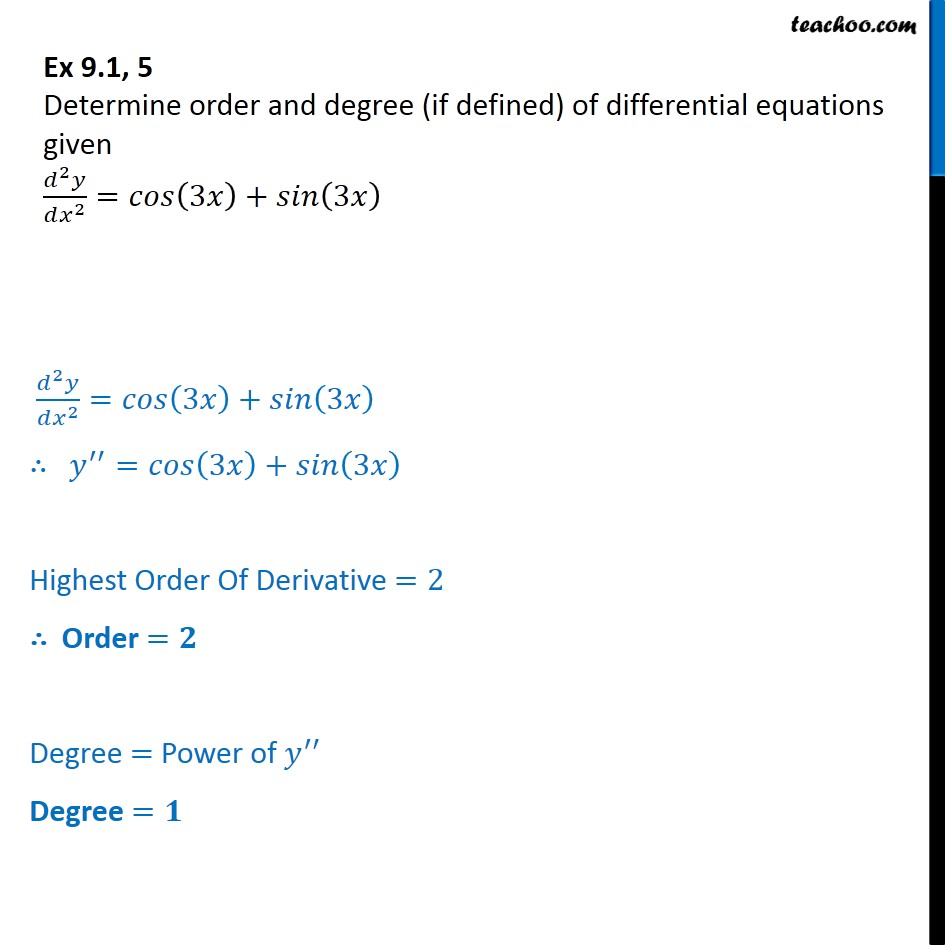Ex 9.1

Chapter 9 Class 12 Differential Equations
Serial order wiseLearn in your speed, with individual attention - Teachoo Maths 1-on-1 Class

### Transcript

Ex 9.1, 5 Determine order and degree (if defined) of differential equations given d2y/dx2 = cos (3x) + sin (3x) d2y = cos (3x) + sin (3x) y" = cos Highest Order Of Derivative =2 Order = Degree = Power of y" Degree =